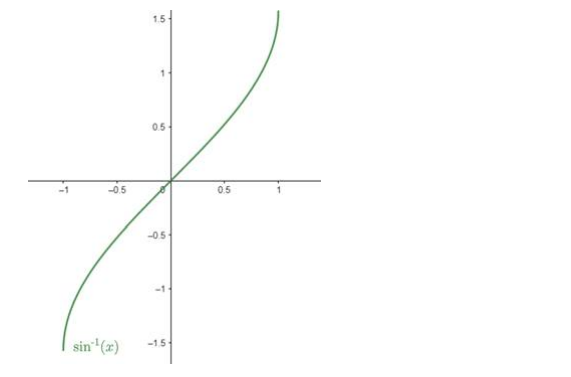# Mark (√) against the correct answer in the following:`
Question:

Mark (√) against the correct answer in the following:

Let $f(x)=\frac{\sin ^{-1} x}{x} .$ Then, dom $(f)=?$

A. $(-1,1)$

B. $[-1,1]$

C. $[-1,1]-\{0\}$

D. none of these

Solution:

Given: $f(x)=\frac{\sin ^{-1} x}{x}$

From f(x), x ≠ 0

Now, domain of $\sin ^{-1} x$ is $[-1,1]$ as the values of $\sin ^{-1} x$ lies between $-1$ and 1 .

We can see that from this graph:Domain of $f(x)=[-1,1]-0$

Hence, B is the correct answer.